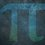# Erdős–Moser Equation Proof (Part $1$ - Setting the Boundaries and Definition)

In number theory, the Erdős–Moser equation is defined as

$1^k + 2^k + ... + m^k = (m + 1)^k$

Of course, a well-known trivial solution is

$1^1 + 2^1 = 3^1$

However, Paul Erdős conjectured that no further solutions exist to the Erdős–Moser equation other than

$1^1 + 2^1 = 3^1$

So, now what?

Well, a solution to find $m$ is

$m^k - (m + 1)^k = - 1 - 2^k$

since

$1^k = 1$

Leo Moser also posed certain constraints on the solutions:

$1$. $\frac{2}{k} = x$, where $x$ is a positive number and that there is no other solution for $m < 10^{1,000,000}$, which Leo Moser himself proved in $1953$.

$2$. $k + 2 < m < 2k$, which was shown in $1966$.

$3$. $lcm (1, 2, ..., 200)$ divides $k$ and that any prime factor of $m + 1$ must be irregular and $> 10,000$, which was shown in $1994$.

$4$. In $1999$, Moser's method was extended to show that $m > 1,485 \times 10^{9,321,155}$ .

$5$. In $2002$, it was shown that $200 < p < 1000$ must divide $k$, where $p$ is all the primes in between.

$6$. In $2009$, it was shown that $\frac{2k}{2m - 3}$ must be a convergent of $In (2)$ - large-scale computation was used to show that $m > 2.7139 \times 10^{1,667,658,416}$.Note by Yajat Shamji
5 months, 3 weeks ago

This discussion board is a place to discuss our Daily Challenges and the math and science related to those challenges. Explanations are more than just a solution — they should explain the steps and thinking strategies that you used to obtain the solution. Comments should further the discussion of math and science.

When posting on Brilliant:

• Use the emojis to react to an explanation, whether you're congratulating a job well done , or just really confused .
• Ask specific questions about the challenge or the steps in somebody's explanation. Well-posed questions can add a lot to the discussion, but posting "I don't understand!" doesn't help anyone.
• Try to contribute something new to the discussion, whether it is an extension, generalization or other idea related to the challenge.

MarkdownAppears as
*italics* or _italics_ italics
**bold** or __bold__ bold
- bulleted- list
• bulleted
• list
1. numbered2. list
1. numbered
2. list
Note: you must add a full line of space before and after lists for them to show up correctly
paragraph 1paragraph 2

paragraph 1

paragraph 2

[example link](https://brilliant.org)example link
> This is a quote
This is a quote
    # I indented these lines
# 4 spaces, and now they show
# up as a code block.

print "hello world"
# I indented these lines
# 4 spaces, and now they show
# up as a code block.

print "hello world"
MathAppears as
Remember to wrap math in $$ ... $$ or $ ... $ to ensure proper formatting.
2 \times 3 $2 \times 3$
2^{34} $2^{34}$
a_{i-1} $a_{i-1}$
\frac{2}{3} $\frac{2}{3}$
\sqrt{2} $\sqrt{2}$
\sum_{i=1}^3 $\sum_{i=1}^3$
\sin \theta $\sin \theta$
\boxed{123} $\boxed{123}$